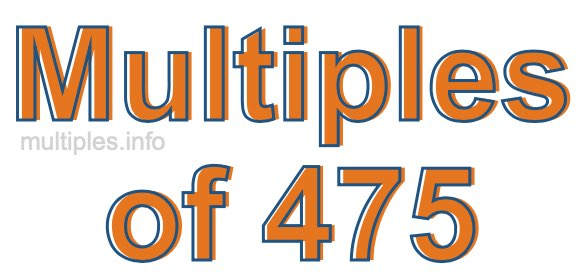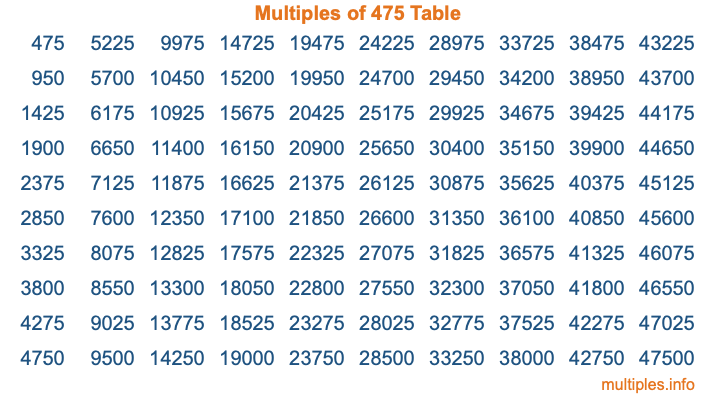Multiples of 475Welcome to the Multiples of 475 page. Here we will first teach you everything you will ever need to know about the multiples of 475, and then give you a study guide summary of everything we taught you to make sure you remember it all. Use this page to look up facts and learn information about the multiples of 475. This page will make you a multiples of four hundred seventy-five expert!

Definition of Multiples of 475
Multiples of 475 are all the numbers that when divided by 475 equal an integer. Each of the multiples of 475 are called a multiple. A multiple of 475 is created by multiplying 475 by an integer.

Therefore, to create a list of multiples of 475, you start with 1 multiplied by 475, then 2 multiplied by 475, then 3 multiplied by 475, and so on for as long as you want. Thus, the list of the first five multiples of 475 is 475, 950, 1425, 1900, and 2375. To see a larger list of multiples of 475, see the printable image of Multiples of 475 further down on this page. We also have a category where you can choose any nth multiple of 475.

Multiples of 475 Checker
The Multiples of 475 Checker below checks to see if any number of your choice is a multiple of 475. In other words, it checks to see if there is any number (integer) that when multiplied by 475 will equal your number. To do that, we divide your number by 475. If the the quotient is an integer, then your number is a multiple of 475.

Is  a multiple of 475?

Least Common Multiple of 475 and ...
A Least Common Multiple (LCM) is the lowest multiple that two or more numbers have in common. This is also called the smallest common multiple or lowest common multiple and is useful to know when you are adding our subtracting fractions. Enter one or more numbers below (475 is already entered) to find the LCM.

Check out our LCM Calculator if you need more details about the Least Common Multiple or if you need the LCM for different numbers for adding and subtraction fractions.

nth Multiple of 475
As we stated above, 475 is the first multiple of 475, 950 is the second multiple of 475, 1425 is the third multiple of 475, and so on. Enter a number below to find the nth multiple of 475.

th multiple of 475

Multiples of 475 vs Factors of 475
475 is a multiple of 475 and a factor of 475, but that is where the similarities end. All postive multiples of 475 are 475 or greater than 475. All positive factors of 475 are 475 or less than 475.

Below is the beginning list of multiples of 475 and the factors of 475 so you can compare:

Multiples of 475: 475, 950, 1425, 1900, 2375, etc.

Factors of 475: 1, 5, 19, 25, 95, 475

As you can see, the multiples of 475 are all the numbers that you can divide by 475 to get a whole number. The factors of 475, on the other hand, are all the whole numbers that you can multiply by another whole number to get 475.

It's also interesting to note that if a number (x) is a factor of 475, then 475 will also be a multiple of that number (x).

Multiples of 475 vs Divisors of 475
The divisors of 475 are all the integers that 475 can be divided by evenly. Below is a list of the divisors of 475.

Divisors of 475: 1, 5, 19, 25, 95, 475

The interesting thing to note here is that if you take any multiple of 475 and divide it by a divisor of 475, you will see that the quotient is an integer.

Multiples of 475 Table
Below is an image of the first 100 multiples of 475 in a table. The table is in chronological order, column by column. The first column has the first ten multiples of 475, the second column has the next ten multiples of 475, and so on.The Multiples of 475 Table is also referred to as the 475 Times Table or Times Table of 475. You are welcome to print out our table for your studies.

Negative Multiples of 475
Although not often discussed or needed in math, it is worth mentioning that you can make a list of negative multiples of 475 by multiplying 475 by -1, then by -2, then by -3, and so on, to get the following list of negative multiples of 475:

-475, -950, -1425, -1900, -2375, etc.

Multiples of 475 Summary
Below is a summary of important Multiples of 475 facts that we have discussed on this page. To retain the knowledge on this page, we recommend that you read through the summary and explain to yourself or a study partner why they hold true.

There are an infinite number of multiples of 475.

A multiple of 475 divided by 475 will equal a whole number.

475 divided by a factor of 475 equals a divisor of 475.

The nth multiple of 475 is n times 475.

The largest factor of 475 is equal to the first positive multiple of 475.

475 is a multiple of every factor of 475.

475 is a multiple of 475.

A multiple of 475 divided by a divisor of 475 equals an integer.

475 divided by a divisor of 475 equals a factor of 475.

Any integer times 475 will equal a multiple of 475.

Multiples of a Number
Here you can get the multiples of another number, all with the same attention to detail as we did for multiples of 475 on this page.

Multiples of
Multiples of 476
Did you find our page about multiples of four hundred seventy-five educational? Do you want more knowledge? Check out the multiples of the next number on our list!